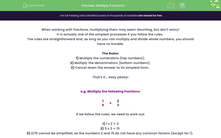# Multiply Fractions

In this worksheet, students will practise multiplying fractions and simplifying answers by finding the Greatest Common Factor (GCF).Key stage:  KS 4

Year:  GCSE

GCSE Subjects:   Maths

GCSE Boards:   Pearson Edexcel, OCR, Eduqas, AQA,

Curriculum topic:   Number, Fractions, Decimals and Percentages

Curriculum subtopic:   Structure and Calculation Fractions

Difficulty level:#### Worksheet Overview

When working with fractions, multiplying them may seem daunting, but don't worry!

It is actually one of the simplest processes if you follow the rules.

The rules are straightforward and, as long as you can multiply and divide whole numbers, you should have no trouble.

The Rules:

1) Multiply the numerators (top numbers);

2) Multiply the denominators (bottom numbers);

3) Cancel down the answer to its simplest form.

That's it... easy peasy!

e.g. Multiply the following fractions:

 1 5
x
 2 3

If we follow the rules, we need to work out:

1) 1 x 2 = 2

2) 5 x 3 = 15

3) 2/15 cannot be simplified, as the numbers 2 and 15 do not have any common factors (except for 1).

Let's see this as the sum:

 1 5
x
 2 3
=
 2 15

Let's try another...

e.g. Multiply the following fractions:

 3 5
x
 5 11

If we follow the rules, we reach:

 3 5
x
 5 11
=
 15 55

If we now look at our new fraction, we need to find the largest number which we can divide both 11 and 55 by.

As the biggest number is 5, this means we divide both the numerator and denominator by 5:

 15 55
=
 15 ÷ 5 55 ÷ 5
=
 3 11

In this activity, you will practise multiplying fractions and simplifying the answer you reach when possible.

### What is EdPlace?

We're your National Curriculum aligned online education content provider helping each child succeed in English, maths and science from year 1 to GCSE. With an EdPlace account you’ll be able to track and measure progress, helping each child achieve their best. We build confidence and attainment by personalising each child’s learning at a level that suits them.

Get started